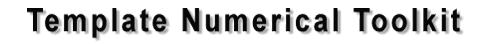Overview TNT consists of an interface and reference implementation of multidimensional arrays for numerical computing. This page lists the components of TNT, togeter with links to external libraries that utilize this interface.
• TNT Data Structures
• C-style arrays
• Fortran-style arrays
• Sparse Matrices
• Vector/Matrix
• TNT utilities
• array I/O
• math routines (hypot(), sign(), etc.)
• Stopwatch class for timing measurements
• Libraries that utilize TNT
• JAMA: a linear algebra library with QR, SVD, Cholesky and Eigenvector solvers.
• old (pre 1.0) TNT routines for LU, QR, and Eigenvalue problems

### TNT Data Structures

• Numerical Arrays (pass-by-reference semantics)

• C-style arrays:

accessed as `A[i][j]`, with `A` as the first element, stored in "row-major" storage order (i.e. right-most dimension varying fastest). This is useful when interfacing with codes written in C or using native "multidimensional" arrays of C/C++.

• Fortran-style arrays:

accessed as `A(i,j)`, with `A(1,1)` as the first element, stored contiguous in memory in "column-major" storage order (i.e. left-most dimension varying fastest). This is useful when translating codes from Fortran by hand or via f2c().

• Sparse Matrices (pass-by-reference semantics)

• Vector/Matrix (pass-by-value semantics)

[Note]: The `Vector/Matrix` classes have been superceded by the `Array` classes but are retained for backward compatibility.